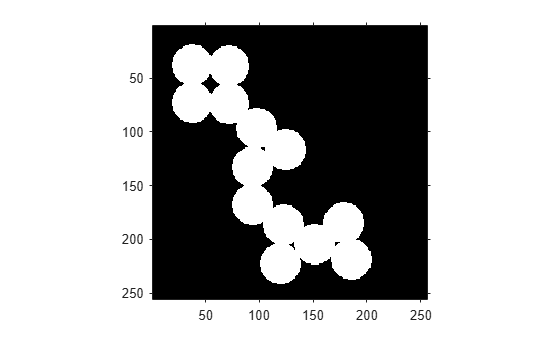# bwarea

Area of objects in binary image

## Syntax

``total = bwarea(BW)``

## Description

example

````total = bwarea(BW)` estimates the area of the objects in binary image `BW`. `total` is a scalar whose value corresponds roughly to the total number of `on` pixels in the image, but might not be exactly the same because different patterns of pixels are weighted differently.```

## Examples

collapse all

Read a binary image and display it.

```BW = imread('circles.png'); imshow(BW)```Calculate the area of objects in the image.

`bwarea(BW)`
```ans = 1.4187e+04 ```

## Input Arguments

collapse all

Binary image, specified as a 2-D numeric or logical matrix. For numeric input, any nonzero pixels are considered to be `1` (`true`).

Example: ```BW = imread('text.png'); L = bwlabel(BW);```

Data Types: `single` | `double` | `int8` | `int16` | `int32` | `int64` | `uint8` | `uint16` | `uint32` | `uint64` | `logical`

## Output Arguments

collapse all

Estimated number of `on` pixels in binary image `BW`, returned as a numeric scalar.

Data Types: `double`

## Algorithms

`bwarea` estimates the area of all of the `on` pixels in an image by summing the areas of each pixel in the image. The area of an individual pixel is determined by looking at its 2-by-2 neighborhood. There are six different patterns, each representing a different area:

• Patterns with zero `on` pixels (area = 0)

• Patterns with one `on` pixel (area = 1/4)

• Patterns with two adjacent `on` pixels (area = 1/2)

• Patterns with two diagonal `on` pixels (area = 3/4)

• Patterns with three `on` pixels (area = 7/8)

• Patterns with all four `on` pixels (area = 1)

Each pixel is part of four different 2-by-2 neighborhoods. This means, for example, that a single `on` pixel surrounded by `off` pixels has a total area of 1.

 Pratt, William K., Digital Image Processing, New York, John Wiley & Sons, Inc., 1991, p. 634.